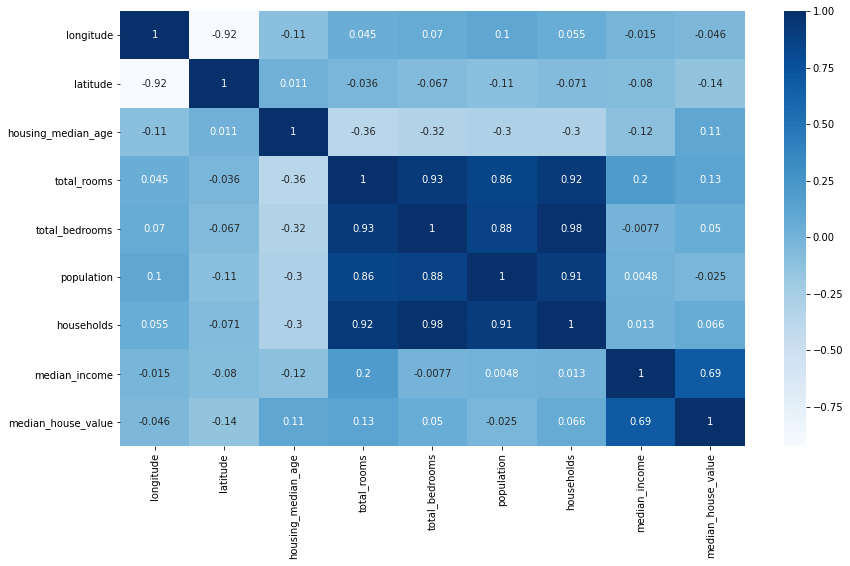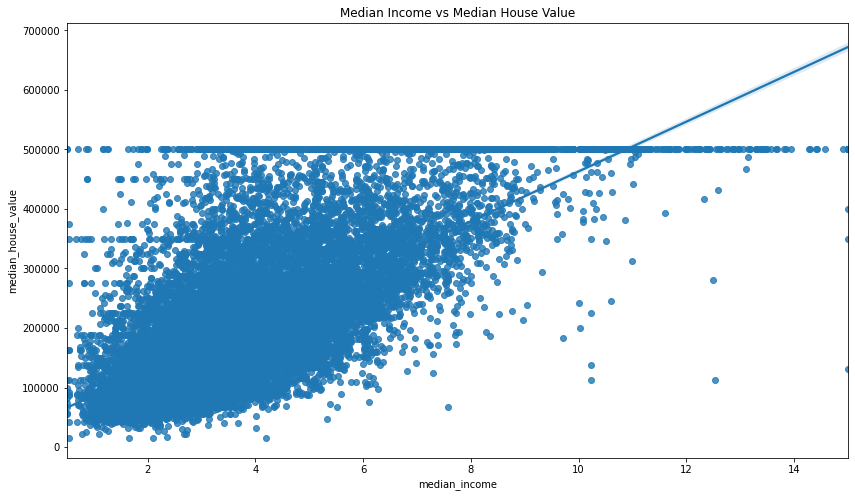Picture by Skylar Kang, Pexels.

The Pearson correlation coefficient, or PCC, is the standard statistical method for computing pairwise or bivariate correlation in Pandas. It’s so commonly used in statistics, that it is often referred to simply as “the correlation coefficient.” Pearson correlation coefficient is exactly the same thing as Pearson’s r, and the Pearson product moment correlation coefficient (PPMCC), it just has several names.

As with other correlation coefficients, Pearson correlation is used to compute the strength of linear correlation between two variables in a dataset. It’s basically the ratio between the covariance of the variables and the product of their standard deviations, and gives a normalised measure of covariance that returns a value between 1 and -1. A value of 1 indicates a perfect positive linear relationship, a value of -1 indicates a perfect negative linear relationship, and a value of 0 indicates no linear relationship.

By interpreting the result of the Pearson correlation coefficient you can tell whether one variable is associated with a change in the other. For example, the price of new cars might be positively correlated with top speed, since most very expensive new cars are supercars or hypercars.

In this tutorial, I’ll explain Pearson correlation and show how you can use it to spot correlations between variables in your dataset using the Pandas `corr()` function.

### Pearson correlation coefficient using corr()

The Pearson correlation coefficient can be easily calculated in Pandas using `corr()`. The `corr()` function is used to compute pairwise correlation coefficients on Pandas dataframe values, and can either calculate them as individual pairs (i.e. top speed and price), or as pairs across an entire dataframe.

Since Pearson correlation coefficient is so widely used by statisticians and data scientists, the `corr()` function is pre-configured with default values to return the Pearson correlation coefficient. However, you can change this to use the similar Spearman rank correlation (or Spearman’s r), or the Kendall Tau correlation coefficient, if you think they better suit your data.

The `corr()` function takes three arguments, all of which are optional. Here’s a quick summary of what they do.

Parameter Description
`method` The `method` parameter defines which of the three correlation coefficient methods to use. The default is `pearson`, which is the Pearson product-moment correlation coefficient. The other two methods are `kendall`, for the Kendall Tau correlation coefficient, and `spearman` for the Spearman's rank correlation coefficient.
`min_periods` The `min_periods` parameter defines the minimum number of observations required to calculate the correlation coefficient. If there are fewer than `min_periods` observations, the correlation coefficient will be set to `NaN`. This currently only works when the `method` parameter is set to `pearson` or `spearman`.
`numeric_only` The `numeric_only` parameter was new in Pandas 1.5.0, so it won't work on earlier releases of Pandas. This parameter defines whether to only calculate the correlation coefficient on numeric columns. If set to `True`, only numeric columns will be used to calculate the correlation coefficient. If set to `False`, all columns will be used to calculate the correlation coefficient. The default is `True`. In a future release, the value of `numeric_only` will change to `False`, so if you don't set it you'll currently get this warning: "FutureWarning: The default value of numeric_only in DataFrame.corr is deprecated. In a future version, it will default to False. Select only valid columns or specify the value of numeric_only to silence this warning." Passing `numeric_only=True` will fix it.

#### Interpreting Pearson’s correlation coefficient

When using `corr(method='pearson')`, or just `corr()`, since `method='pearson'` is the default, the resulting correlation matrix will have a value for every pair of columns in the original DataFrame.

The value will be a number between -1 and 1, where 1 is a perfect positive linear relationship, 0 is no linear relationship, and -1 is a perfect negative linear relationship. Different authors use slightly different interpretations of the coefficients, but they’re generally very similar to the ones below.

Pearson's r value Strength of relationship
0 No linear relationship
0.1 to 0.3 Weak linear relationship
0.3 to 0.5 Moderate linear relationship
0.5 to 0.7 Strong linear relationship
0.7 to 1 Very strong linear relationship
-0.1 to -0.3 Weak negative linear relationship
-0.3 to -0.5 Moderate negative linear relationship
-0.5 to -0.7 Strong negative linear relationship
-0.7 to -1 Very strong negative linear relationship

To get started, open a new Jupyter notebook and import the Pandas library. You only need this to calculate correlation coefficients in Pandas, but to cover some more advanced topics we’ll also import the Matplotlib and Seaborn data visualisation packages, and the Scipy statistical analysis package.

``````import pandas as pd
import matplotlib.pyplot as plt
import seaborn as sns
from scipy.stats import pearsonr
``````

### Import a dataset

Then, either create a dummy dataset of data with linear relationships between the variables, or import a dataset into Pandas from a CSV file. I’m using a house price dataset, as this contains several correlated variables.

``````df = pd.read_csv('https://raw.githubusercontent.com/flyandlure/datasets/master/housing.csv')
``````
0 1 2 3 4
longitude -122.23 -122.22 -122.24 -122.25 -122.25
latitude 37.88 37.86 37.85 37.85 37.85
housing_median_age 41.0 21.0 52.0 52.0 52.0
total_rooms 880.0 7099.0 1467.0 1274.0 1627.0
total_bedrooms 129.0 1106.0 190.0 235.0 280.0
population 322.0 2401.0 496.0 558.0 565.0
households 126.0 1138.0 177.0 219.0 259.0
median_income 8.3252 8.3014 7.2574 5.6431 3.8462
median_house_value 452600.0 358500.0 352100.0 341300.0 342200.0
ocean_proximity NEAR BAY NEAR BAY NEAR BAY NEAR BAY NEAR BAY

### Calculate the Pearson correlation for a pair of columns

To calculate the Pearson correlation for a pair of columns, you can append the `.corr()` method to the first column and pass the second column as an argument. If we do this for the `median_income` and `median_house_value` columns we get back a PCC or r of 0.6880, which according to our interpretation in the lookup table denotes a strong linear correlation. That means, samples with a higher `median_income` generally had a higher `median_house_value`.

``````df['median_income'].corr(df['median_house_value'])
``````
``````0.688075207958548
``````

### Calculate the Pearson correlation for all columns in the dataframe

To calculate the Pearson correlation coefficient for every pair of values in the dataframe, you can simply append the `corr()` method to the end of the dataframe object. The resulting dataframe, or matrix, will have the correlation coefficient for every pair of columns in the dataframe. To avoid getting a `FutureWarning`, you’ll want to set `numeric_only=True` in the `corr()` method.

``````df.corr(numeric_only=True)
``````
longitude latitude housing_median_age total_rooms total_bedrooms population households median_income median_house_value
longitude 1.000000 -0.924664 -0.108197 0.044568 0.069608 0.099773 0.055310 -0.015176 -0.045967
latitude -0.924664 1.000000 0.011173 -0.036100 -0.066983 -0.108785 -0.071035 -0.079809 -0.144160
housing_median_age -0.108197 0.011173 1.000000 -0.361262 -0.320451 -0.296244 -0.302916 -0.119034 0.105623
total_rooms 0.044568 -0.036100 -0.361262 1.000000 0.930380 0.857126 0.918484 0.198050 0.134153
total_bedrooms 0.069608 -0.066983 -0.320451 0.930380 1.000000 0.877747 0.979728 -0.007723 0.049686
population 0.099773 -0.108785 -0.296244 0.857126 0.877747 1.000000 0.907222 0.004834 -0.024650
households 0.055310 -0.071035 -0.302916 0.918484 0.979728 0.907222 1.000000 0.013033 0.065843
median_income -0.015176 -0.079809 -0.119034 0.198050 -0.007723 0.004834 0.013033 1.000000 0.688075
median_house_value -0.045967 -0.144160 0.105623 0.134153 0.049686 -0.024650 0.065843 0.688075 1.000000

### Visualise the correlation coefficients with a heatmap

When examining a dataset it’s often easier to visualise the correlations using Seaborn, or a similar data visualisation library. Visualising the correlations via a heatmap is my preferred technique and is easily done by using just a Seaborn function and a Matplotlib function to adjust the figure size.

``````plt.figure(figsize=(14,8))
sns.heatmap(df.corr(numeric_only=True), annot=True, cmap='Blues')
``````
``````<AxesSubplot:>
``````### Visualising correlations between pairs of columns

There are various ways to visualise correlations between pairs of variables. A quick way to view the correlations of a single pair is to use the Seaborn `regplot()` function. This takes three arguments comprising the `x` and `y` columns you want to plot, and the `data` from the dataframe.

``````plt.figure(figsize=(14,8))
sns.regplot(x='median_income', y='median_house_value', data=df)
plt.title('Median Income vs Median House Value')
``````
``````Text(0.5, 1.0, 'Median Income vs Median House Value')
``````### Calculate the statistical significance of the correlation coefficient

To determine whether the correlation coefficient is statistically significant or not you can use the `pearsonr()` method from `scipy.stats`. This returns does much the same as the Pandas `corr()` function, in that it also returns the correlation coefficient, but crucially, it provides the p-value that Pandas does not.

The p-value is the probability that the correlation coefficient is not statistically significant. If the p-value is less than 0.05 then the correlation coefficient is statistically significant. We get a very low p value, so the correlation coefficient is statistically significant.

``````pearsonr(df['median_income'], df['median_house_value'])
``````
``````(0.6880752079585477, 0.0)
``````

By contrast, if you use Scipy to calculate the Pearson correlation coefficient and p value of the `housing_median_age` and `median_house_value` columns you get back a higher p value, indicating that the result is not statistically significant.

``````pearsonr(df['housing_median_age'], df['median_house_value'])
``````
``````(0.10562341249320992, 2.7618606761502365e-52)
``````

Matt Clarke, Sunday, November 20, 2022

Matt Clarke Matt is an Ecommerce and Marketing Director who uses data science to help in his work. Matt has a Master's degree in Internet Retailing (plus two other Master's degrees in different fields) and specialises in the technical side of ecommerce and marketing.